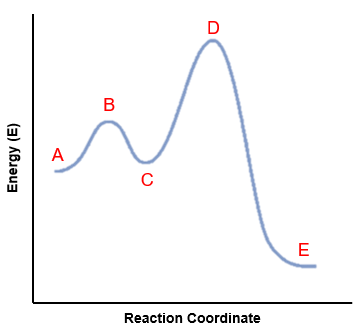# Problem: The activation energy for the reaction associated with the figure shown isa. D minus Eb. D minus Cc. D minus Ad. B minus Ae. B minus C

###### FREE Expert Solution

We’re being asked to identify the activation energy for the reaction associated with the figure below.Recall that an energy diagram is usually read from left to right

The components of a two-step energy diagram are:

87% (160 ratings)###### Problem Details

The activation energy for the reaction associated with the figure shown isa. D minus E
b. D minus C
c. D minus A
d. B minus A
e. B minus C